# Polyhedra in Knots

Celtic knots, or interlace, are a beautiful, ancient art form with interesting symmetries and topological properties: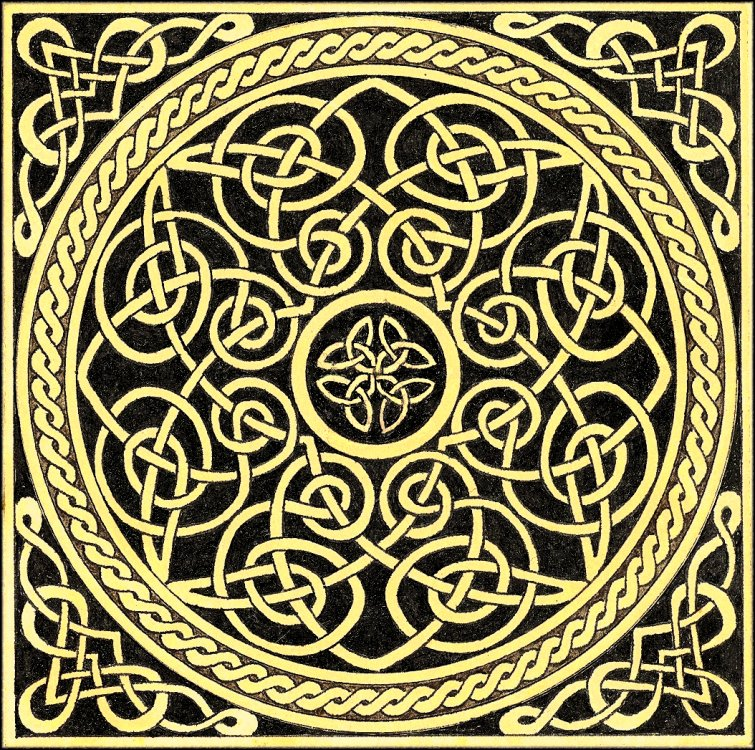Every planar graph corresponds with a Celtic knotwork, and vice-versa. The Mercat procedure shows how to convert a graph into knotwork (see linked page for details); the procedure is precise enough even to be implemented as a computer program.

Having discovered Celtic knots, I wanted to discover how various graphs looked when converted by hand into Celtic knots. I chose a straightforward collection of graphs: the five Platonic solids, considered as vertices joined by edges.

It was a bit of a struggle for the imagination at first, chasing threads around the corners of polyhedra and visualizing where they would end up. The problem was only partially solved by unfolding the polyhedra, because then threads that vanished off one side of the figure would appear on another in a seemingly different orientation. Eventually, I discovered that I could represent the edges of the graph and their "shares vertex" relationships as graphs themselves. These graphs were all planar, and simplified the Mercat procedure terrifically.

I picked from the list of regular polyhedra at random, discovering a pattern: all of their knotwork seemed to consist solely of overlapping circles:

```tetrahedron: 3 circles
cube: 4 circles
octahedron: ___
dodecahedron: 6 circles
icosahedron: __
```

What's the pattern? Imagine my surprise when I drew out the knotwork for the rest and found out that the pattern was:

```tetrahedron: 3 circles
cube: 4 circles
octahedron: 4 circles (!)
dodecahedron: 6 circles
icosahedron: 6 circles (!)
```

The knotwork for the cube and octahedron was identical, as was the knotwork for the dodecahedron and the icosahedron! In retrospect, these relationships are less mysterious in light of dual polyhedra: the cube and octahedron are dual pairs, as are the dodecahedron and the icosahedron. This means, in part, that if you draw lines to connect the faces of one shape, you get the dual shape. (The tetrahedron, on its own, is self-dual.)

(I wonder if we can state the proof that there are only five platonic solids in terms of the number of circles that a hypothetical platonoic solid would have in its knotwork, and how those circles would overlap?)

What follows is a gallery of my handwritten drawings on the subject, because I think they look interesting, and might be enjoyable or useful to see:

Tetrahedron. Here, I use my abstract method to draw the knotwork for the tetrahedron. Each node in the graph represents a pair of faces (an edge of the polyhedron), and each edge in the graph represents a shared-vertex relationship. The edges are oriented at various multiples of 45 degrees, as they must be lined up in the final knotwork.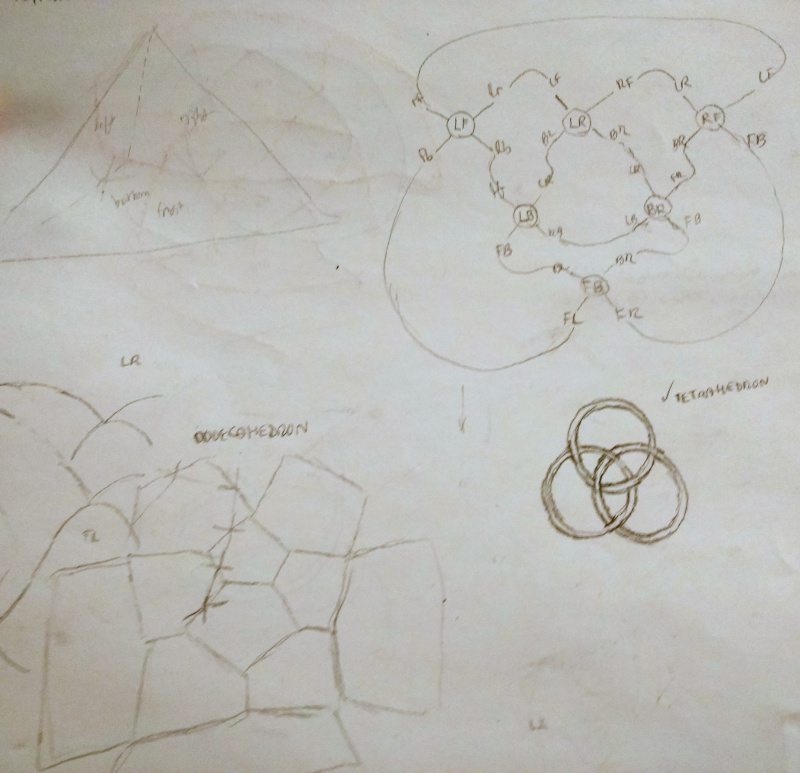Cube. The cube was the first shape I attempted, actually. You can see in the bottom left, I attempted to visualize the movements of the threads in 3D. When I looked for a simpler method, I tried unfolding the cube and having the threads "wrap around" as needed (upper-left cross-like figure). The apparent orientation changes (in the 2D unfolding) made this method a bit difficult. On the right right, I begin to wonder whether I can represent the knotwork as overlapping circles.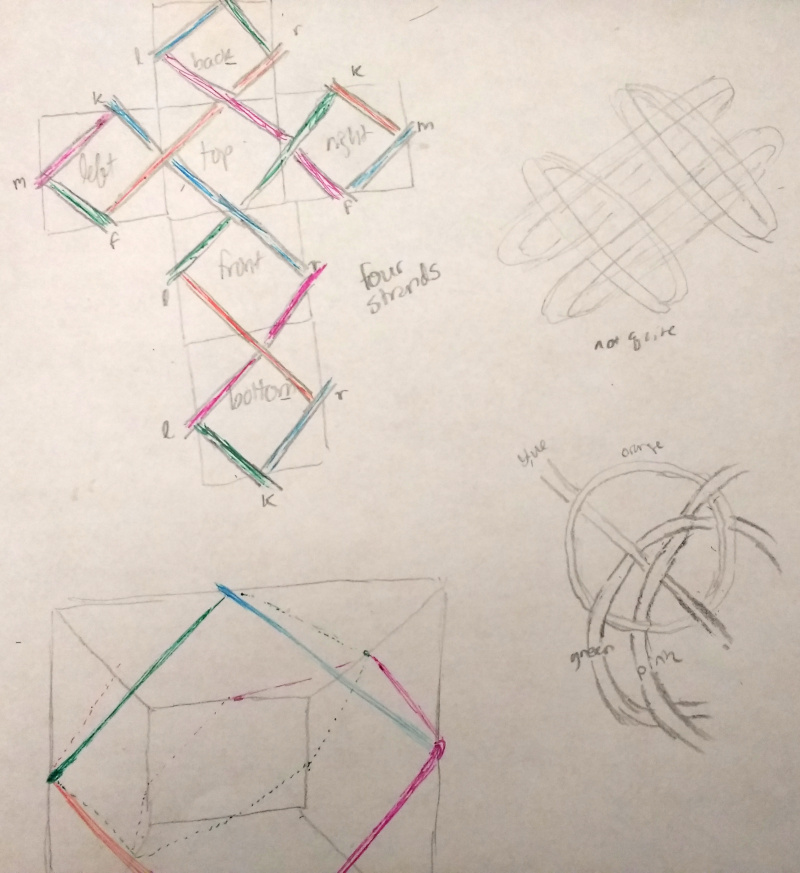Cube #2. Following my initial hunch about overlapping circles…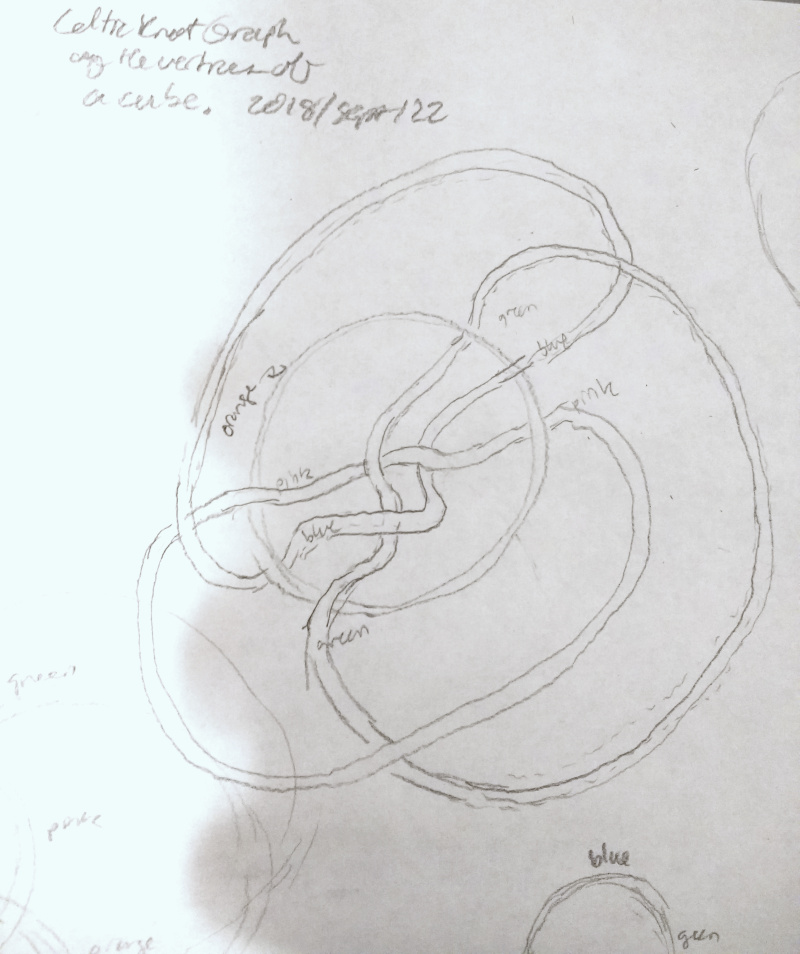…I eventually succeed at drawing the cube's knotwork (four interlocking circles in upper-right of image below), using the abstract method I developed on the simpler tetrahedron (note some stray tetrahedron attempts around the bottom-left periphery). I rather like the teardrop-shaped aesthetic of the middle incarnation, but the four interlocking circles are more symmetric.Dodecahedron. The dodecahedron was a real challenge, moving from the planar graph shown here (with all its intricate thread crossings, two per edge)…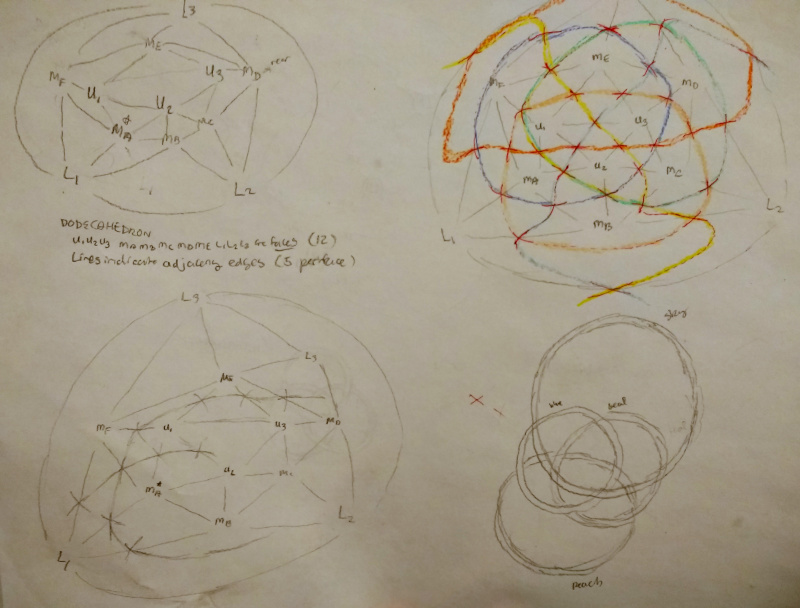… to the final, stunning result: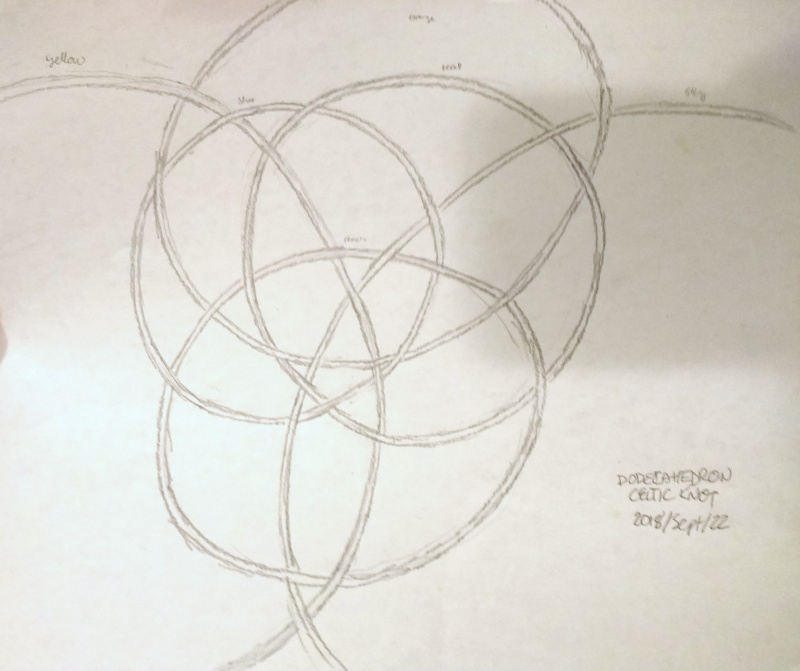Icosahedron I initially attempted the icosahedron's knotwork using the "unfolding" method with its bewildering wrapped threads: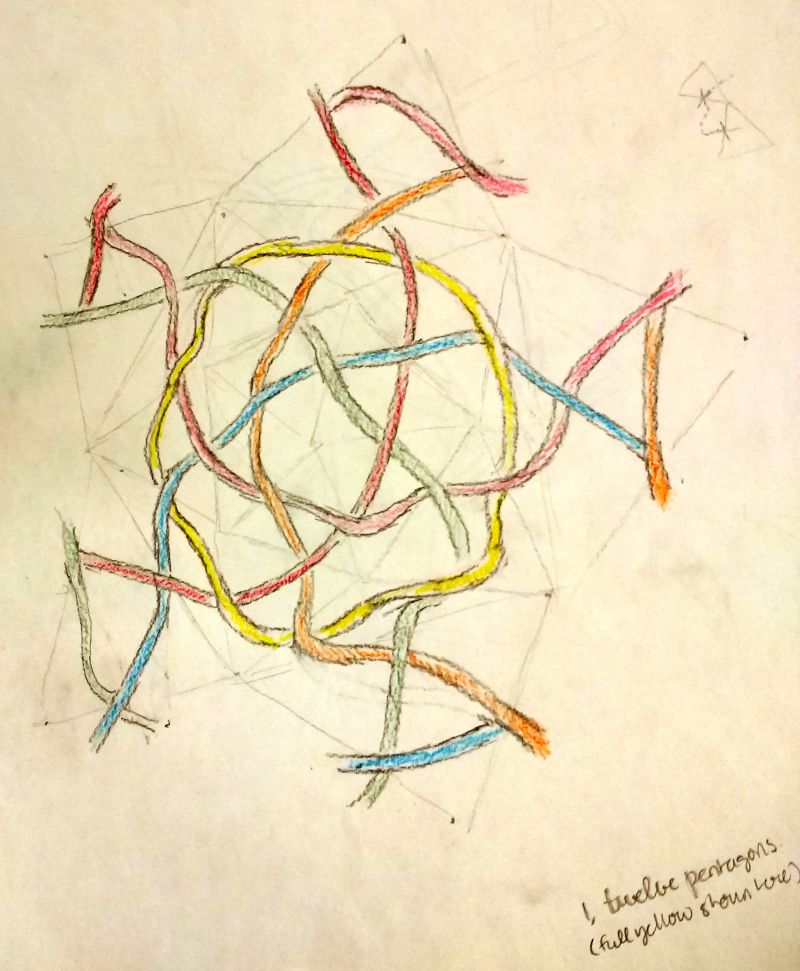Eventually, I discovered the abstract method (and the duality principle), and the rest is exactly the same as for the dodecahedron, shown here again (notice the star shape in the middle of the two figures here? They're overflowing with star-shaped intersections of threads if you look!):Created: 2018-09-22 Sat 21:49

Validate